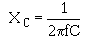Capacitive Reactance alternating current has been the normal circuit resistance present in any conductor. However, capacitors themselves offer a very real opposition to current flow. This opposition arises from the fact that, at a given voltage and frequency, the number of electrons which go back and forth from plate to plate is limited by the storage ability-that is, the capacitance-of the capacitor.">Custom SearchCAPACITIVE REACTANCE So far you have been dealing with the capacitor as a device which passes ac and in which the only opposition to the alternating current has been the normal circuit resistance present in any conductor. However, capacitors themselves offer a very real opposition to current flow. This opposition arises from the fact that, at a given voltage and frequency, the number of electrons which go back and forth from plate to plate is limited by the storage ability-that is, the capacitance-of the capacitor. As the capacitance is increased, a greater number of electrons change plates every cycle, and (since current is a measure of the number of electrons passing a given point in a given time) the current is increased. Increasing the frequency will also decrease the opposition offered by a capacitor. This occurs because the number of electrons which the capacitor is capable of handling at a given voltage will change plates more often. As a result, more electrons will pass a given point in a given time (greater current flow). The opposition which a capacitor offers to ac is therefore inversely proportional to frequency and to capacitance. This opposition is called CAPACITIVE REACTANCE. You may say that capacitive reactance decreases with increasing frequency or, for a given frequency, the capacitive reactance decreases with increasing capacitance. The symbol for capacitive reactance is XC. Now you can understand why it is said that the XC varies inversely with the product of the frequency and capacitance. The formula is:Where: XC is capacitive reactance in ohms f is frequency in Hertz C is capacitance in farads p is 6.28 (2 X 3.1416) The following example problem illustrates the computation of X C.Q.9 What is the term for the opposition that a capacitor presents to ac? Q.10 What is the formula used to compute this opposition? Q.11 What happens to the value of XC as frequency decreases? Q.12 What happens to the value of XC as capacitance increases?Integrated Publishing, Inc. - A (SDVOSB) Service Disabled Veteran Owned Small Business